# Physics - Electromagnetism - Electrical resistivity and conductivity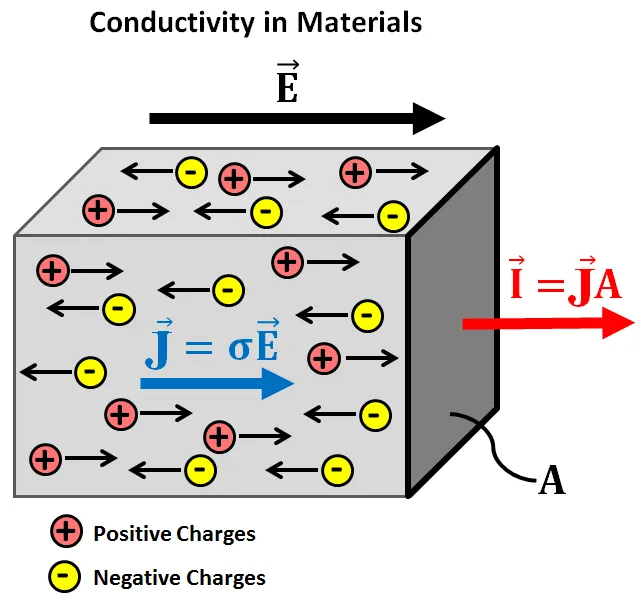Source

## Introduction

Hello it's a me again Drifter Programming!

Today we continue with Electromagnetism to get into Electrical resistivity and conductivity!

All the mathematical equations will be drawn using quicklatex!

So, without further do, let's get straight into it!

## Electrical resistivity of materials

First of all Electrical resistivity that we will talk about should NOT be confused with electric resistance that we will talk about next time!! (but they actually are the same thing, but covered from another respective)

The current density J inside of a conductor depends on the electric field E and the material properties. Generally this dependence is very complex, but in special cases like metals the current density J is proportional to the electric field E. In that special case the ratio of the magnitudes of E and J is constant. By supposing that this is true for every case we start defining new things.

The electrical resistivity (or special resistance) of a material is noted as ρ and equal to the ratio of the electric field E by the current density J.

That way the equation for electrical resistivity ρ is: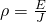The higher the resistivity, the stronger is the electric field required and the smaller the electric current density produced by the electric field.

Electrical resistivity ρ is calculated in: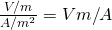where 1 V/A is noted as 1 Ω (ohm) and so ρ is calculated in:

Ω*m (ohm-meter)

something that we will cover more next time!

An ideal conductor would have a resistivity "zero", whilst a ideal insulator would have "infinite" resistivity, but of course there is nothing "perfect" like that in nature!

Metals and Alloys have the smallest value of electrical resistivity and so are great conductors. The special resistance of insulators is much much higher then the resistivity of metals and alloys by a enormous factor of 10^20+!

Here's a table of electrical resistivities:Source

You can see that the metals and alloys have very small resistivities in the order of 10^-8 up to 10^-6, whilst insulators have 10^10 up to 10^17 resistivity!

Worth noting is that Silicon, which is an popular semiconductor, has a resistivity of around 10^2 that puts it into a sweet spot in the center of the "scale". This shows us why semiconductors act like both depending on the situation!

Also note that:

Good conductors of electricity are mostly also great conductors of heat! On the other hand insulators of electricity are mostly also bad conductors of heat. This is true, because heat is also related with the electrons motion!

Semiconductors are special not only because of the electrical resistivity, but because they are also affected by changes in temperature (heat) and also by small quantities of mixtures!

## Electrical conductivity

The ratio of J and E at constant temperature was discovered by G.S. Ohm and that's why the equation that we saw previously is called Ohm's law.

A material that obeys Ohm's law sufficiently is called an ohmic conductor. On the other hand, materials that have a high deviation/difference to Ohm's law are non-ohmic conductors.

Of course this law again applies to ideal materials!

Some info from wikipedia.

The opposite ratio J/E is called electrical conductivity and shows us how conductive a material is! Good conductors of electricity of course have a high value of electrical conductivity, whilst insulators will have smaller values. (similarly we can also define thermal conductivity)

## Thermal coefficient of electrical resistivity

The electrical resistivity of a metal conductor mostly increases when we increase the temperature. This of course means that the electrical conductivity of a conductor decreases when increasing the temperature. This can all be understand better if you think of the motion of the electrons. With higher temperature the motion is even more randomly, making it more difficult to orient them to follow one specific direction.

For a small range of temperatures (approximately 0 up to 100 °C) the electrical resistivity of a metal can be calculated approximately using the equation: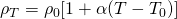where:

• ρ0 is the electrical resistivity for some reference temperature T0
• ρT is the electrical resistivity at a temperature T
• α is the so called Thermal coefficient of electrical resistivity

Here a table with some of those coeffients: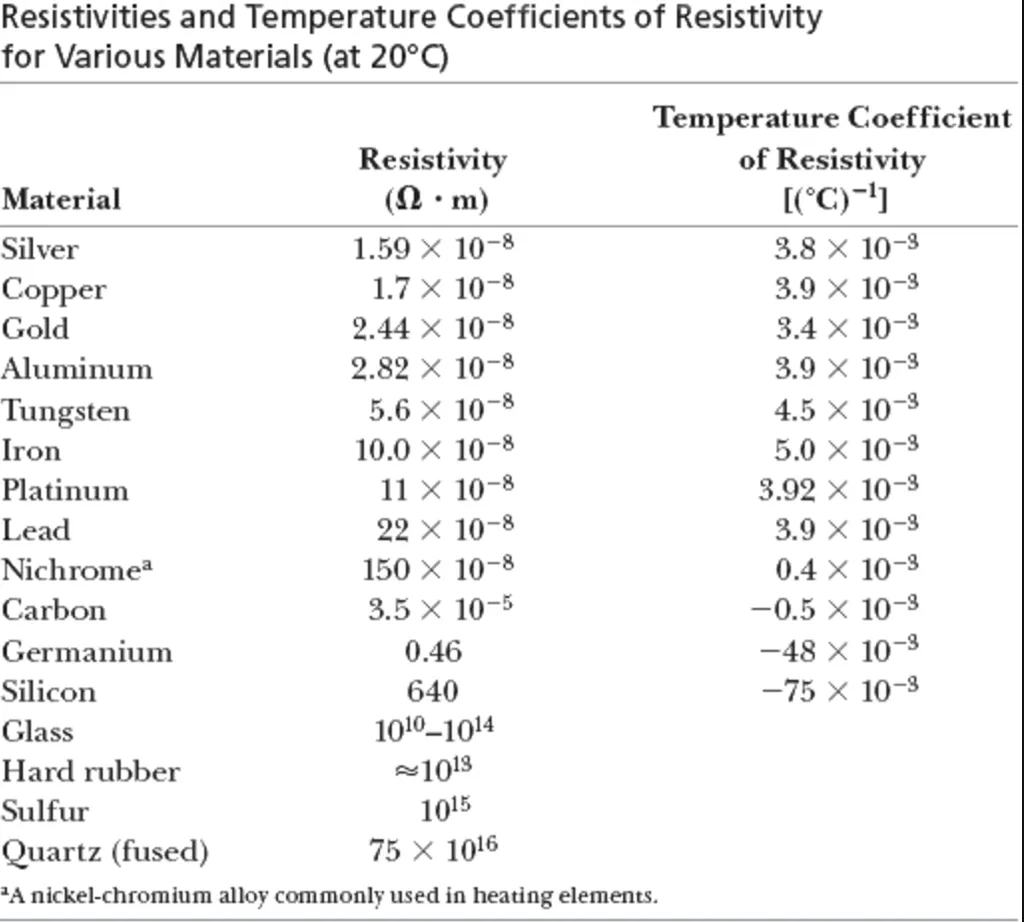Source

It's worth noting that Carbon has a negative coefficient which means that the special resistance is decreasing when the temperature increases!

The resistance of a semiconductors decreases drastically when the temperature increases. That's why a specific small crystal semiconductor called thermistor is used as a sensor of temperature! Other materials like carbon and platinum are also used in thermometers!

## Hyperconductivity

Something interesting is a phenomenon called hyperconductivity which happens for some metal alloys and acids. While the temperature is decreasing, the special resistance is at first also decreasing smoothly, something which is similar to every other metal. But, after a critical temperature Tc we observe a phase transition and the electrical resistance nullifies (changes to zero) sharply! Something which of course means that the electric current flowing through such a material keeps on going, without the need of an electric field E!

But, don't be happy! This phenomenon was observed at temperatures of 4.2K, 20K, 77K and the most recent value 125K (Kelvin) which of course is -148.15 °C! So, getting into room temperature is not far away :P

The discovery of some mixture which gets hyperconductive in a more reasonable temperature would bring a number of positive consequences which would of course help with the energy distribution around the globe!

Intro

Physics Introduction -> what is physics?, Models, Measuring

Vector Math and Operations -> Vector mathematics and operations (actually mathematical analysis, but I don't got into that before-hand :P)

Classical Mechanics

Velocity and acceleration in a rectlinear motion -> velocity, accelaration and averages of those

Rectlinear motion with constant accelaration and free falling -> const accelaration motion and free fall

Rectlinear motion with variable acceleration and velocity relativity -> integrations to calculate pos and velocity, relative velocity

Rectlinear motion exercises -> examples and tasks in rectlinear motion

Position, velocity and acceleration vectors in a plane motion -> position, velocity and accelaration in plane motion

Projectile motion as a plane motion -> missile/bullet motion as a plane motion

Smooth Circular motion -> smooth circular motion theory

Plane motion exercises -> examples and tasks in plane motions

Force and Newton's first law -> force, 1st law

Mass and Newton's second law -> mass, 2nd law

Newton's 3rd law and mass vs weight -> mass vs weight, 3rd law, friction

Applying Newton's Laws -> free-body diagram, point equilibrium and 2nd law applications

Contact forces and friction -> contact force, friction

Dynamics of Circular motion -> circular motion dynamics, applications

Object equilibrium and 2nd law application examples -> examples of object equilibrium and 2nd law applications

Contact force and friction examples -> exercises in force and friction

Circular dynamic and vertical circle motion examples -> exercises in circular dynamics

Electromagnetism

Getting into Electromagnetism -> electromagnetim, electric charge, conductors, insulators, quantization

Coulomb's law with examples -> Coulomb's law, superposition principle, Coulomb constant, how to solve problems, examples

Electric fields and field lines -> Electric fields, Solving problems around Electric fields and field lines

Electric dipoles -> Electric dipole, torque, potential and field

Electric charge and field Exercises -> examples in electric charges and fields

Electric flux and Gauss's law -> Electric flux, Gauss's law

Applications of Gauss's law (part 1) -> applying Gauss's law, Gauss applications

Applications of Gauss's law (part 2) -> more Gauss applications

Electric flux exercises -> examples in electric flux and Gauss's law

Electric potential energy -> explanation of work-energy, electric potential energy

Calculating electric potentials -> more stuff about potential energy, potential, calculating potentials

Millikan's Oil Drop Experiment -> Millikan's experiment, electronvolt

Cathode ray tubes explained using electric potential -> cathode ray tube explanation

Electric potential exercises (part 1) -> applications of potential

Electric potential exercises (part 2) -> applications of potential gradient, advanced examples

Capacitors (Condensers) and Capacitance -> Capacitors, capacitance, calculating capacitance

How to solve problems around Capacitors -> combination, solving problems, simple example

Electric field energy and density -> Electric field energy, energy density

Dielectric materials -> Dielectrics, dielectric constant, permittivity and strength, how to solve problems

Electric capacitance exercises -> examples in capacitance, energy density and dielectrics

Electric current -> Electric current, current density

And this is actually it for today!

Next time I will cover Electric Resistance (easy to confuse with today's topic!).

Bye!

H2
H3
H4
3 columns
2 columns
1 column